Calculate the integral of a parabola is a problem related to the calculation of an area. The problem of calculating the area of a parabolic segment was solved by Archimedes.

The focus changed when mathematicians tried to calculate the area under the graph of a function. This lead to the use (and later the definition) of the concept of integral.

Following Leibniz, we use this symbol to represent the integral:Our interest now is to calculate the area under a parabola (a quadratic function, a polynomial function of degree 2)Some areas are positive and some are negative:This problem was already solved in Cavalieri's time (who could solve the integral for several power functions).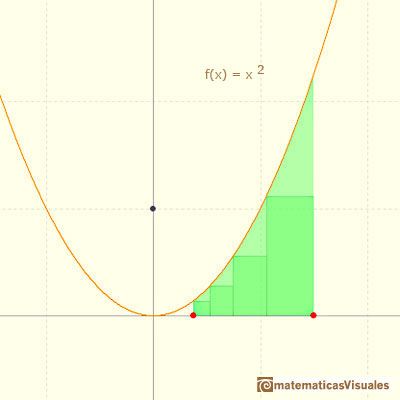One important approach is to use rectangles to approximate the area and then take a limit with the bases of these rectangles tend to zero. When this concept is defined formally this is the Riemann integral.The integral concept is associate to the concept of area. We began considering the area limited by the graph of a function and the x-axis between two vertical lines.If we consider the lower limit of integration a as fixed and if we can calculate the integral for different values of the upper limit of integration b then we can define a new function: an indefinite integral of f.

In this intuitive approach we only use rectangles of equal bases. We can see how using rectangles we can approach the area: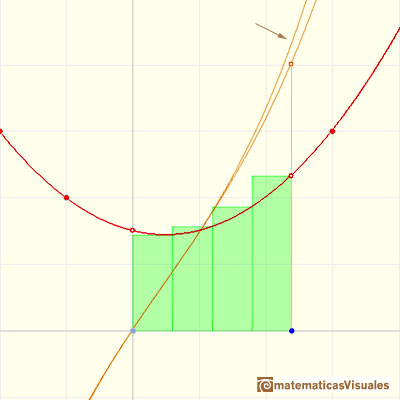Taking two different values for the height of these rectangles we can get two different approximations:If we use more and more rectangles the approximations becomes better and better: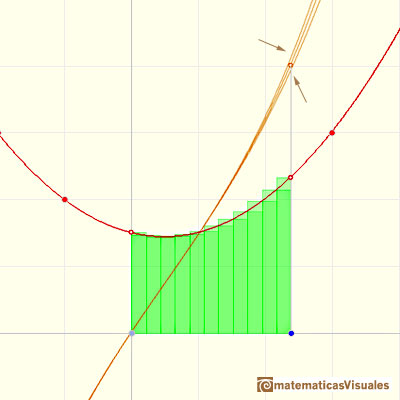We say that quadratic functions are integrable functions because we can calculate an indefinite integral of a parabola.

An integral function of a quadratic function is a polynomial of degree 3.If we change the lower limit of integration, the integral function goes up and down but it does not change its shape. (Why?)

Changing we lower limit of integration we get different integral functions but they are all the same up to a constant (vertical translation).When we integrate a polynomial of degree 1 we get a polynomial of degree 2 and if we integrate a polynomial of degree 2 we get a polynomial of degree 3. When we integrate these functions the result is a polynomial of degree one more than the original function.

Remember that when we derive a quadratic function the result is one less than the original function (a polynomial of degree 1).

These results are related to the Fundamental Theorem of Calculus.

Now we are going to study the average value of a function in this case of quadratic functions.

The average value of a function f(x) over the interval [a,b] is given by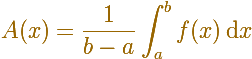The idea is that the area under the function (positive or negative) ...... is the same as the area of a rectangle whose height is the average value.As Quadratic Functions are continuous, they are special cases of the Mean Value Theorem for integrals: If f(x) is a continuous function over the interval [a,b] then there is a number c in [a,b] such that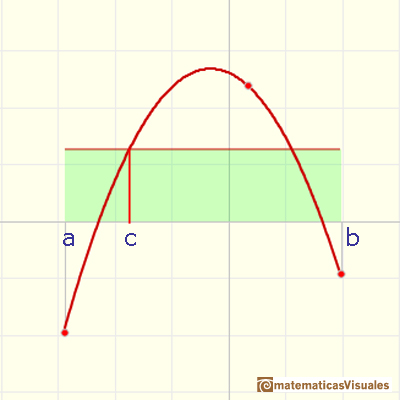REFERENCES

Michael Spivak, Calculus, Third Edition, Publish-or-Perish, Inc.
Tom M. Apostol, Calculus, Second Edition, John Willey and Sons, Inc.Archimedes show us in 'The Method' how to use the lever law to discover the area of a parabolic segment.As an introduction to Piecewise Linear Functions we study linear functions restricted to an open interval: their graphs are like segments.A piecewise function is a function that is defined by several subfunctions. If each piece is a constant function then the piecewise function is called Piecewise constant function or Step function.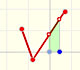A continuous piecewise linear function is defined by several segments or rays connected, without jumps between them.The Fundamental Theorem of Calculus tell us that every continuous function has an antiderivative and shows how to construct one using the integral.The Second Fundamental Theorem of Calculus is a powerful tool for evaluating definite integral (if we know an antiderivative of the function).The integral of power functions was know by Cavalieri from n=1 to n=9. Fermat was able to solve this problem using geometric progressions.The integral concept is associate to the concept of area. We began considering the area limited by the graph of a function and the x-axis between two vertical lines.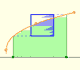Monotonic functions in a closed interval are integrable. In these cases we can bound the error we make when approximating the integral using rectangles.If we consider the lower limit of integration a as fixed and if we can calculate the integral for different values of the upper limit of integration b then we can define a new function: an indefinite integral of f.The derivative of a lineal function is a constant function.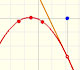The derivative of a quadratic function is a linear function, it is to say, a straight line.The derivative of a cubic function is a quadratic function, a parabola.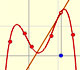Lagrange polynomials are polynomials that pases through n given points. We use Lagrange polynomials to explore a general polynomial function and its derivative.If the derivative of F(x) is f(x), then we say that an indefinite integral of f(x) with respect to x is F(x). We also say that F is an antiderivative or a primitive function of f.Two points determine a stright line. As a function we call it a linear function. We can see the slope of a line and how we can get the equation of a line through two points. We study also the x-intercept and the y-intercept of a linear equation.Power with natural exponents are simple and important functions. Their inverse functions are power with rational exponents (a radical or a nth root)Polynomials of degree 2 are quadratic functions. Their graphs are parabolas. To find the x-intercepts we have to solve a quadratic equation. The vertex of a parabola is a maximum of minimum of the function.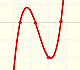Polynomials of degree 3 are cubic functions. A real cubic function always crosses the x-axis at least once.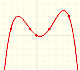We can consider the polynomial function that passes through a series of points of the plane. This is an interpolation problem that is solved here using the Lagrange interpolating polynomial.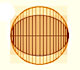In his book 'On Conoids and Spheroids', Archimedes calculated the area of an ellipse. We can see an intuitive approach to Archimedes' ideas.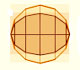In his book 'On Conoids and Spheroids', Archimedes calculated the area of an ellipse. It si a good example of a rigorous proof using a double reductio ad absurdum.Kepler used an intuitive infinitesimal approach to calculate the area of a circle.Kepler was one mathematician who contributed to the origin of integral calculus. He used infinitesimal techniques for calculating areas and volumes.Studying the volume of a barrel, Kepler solved a problem about maxima in 1615.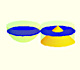Using Cavalieri's Principle we can calculate the volume of a sphere.